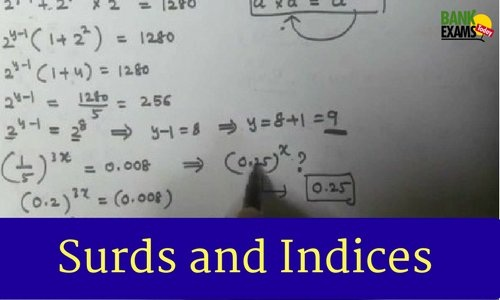# Surds and Indices : Rules explained with Examples### a) Surds :

The surd is represented with symbol √ .The symbol √ is equals to ½.
If we have a number and we are not able to obtain its root. It is called a surd.
The roots of those numbers which cannot be exactly obtained.

Like : √2,√3,√5,√6,√7 and so on.

Mixed Surds : A surd that /which we get by multiplying a surd and a rational number together are called mixed surd.

Like : 7√2,4√3,2√5,6√6,3√7

### Properties of Surds### Rules of addition for surds.

• Two similar surds can be added.
Like : √5+√5 = 2√5 , 2√3+5√3 = 7√3
• Two dissimilar surds cannot be added.
Like : 4√2,2√5,3√6

### b) Indices :

if ‘n’ is a positive integer and ‘a’ is a real number then
we write x×x×x×x×x = xᶯ .here x is the base and n is the power of x.### 3)